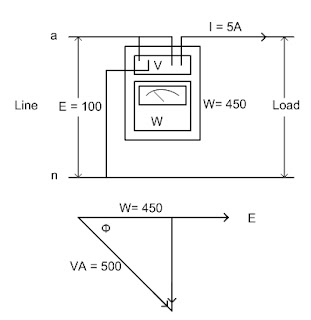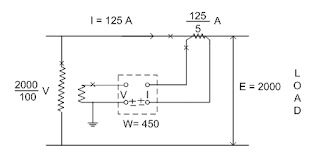### Measurement of AC Power

Instrument Transformer and Power Management (P1) Course
Chapter 4: Power Measurement in 1-ph Circuits
4.1 Measurement of AC Power
Information :
Electrical power is the rate at which electrical energy is transferred or expanded.
Power in an a.c. circuit is classified as either apparent, real or reactive. The formula 10WS their relationship for single phase circuit are shown in the last section.
As noted from the formula, apparent power can be calculated directly from voltage and current measurement. To compute real or reactive power from voltage and current measurements, the phase angle between the voltage and current must also known. Real power is measured directly by a wattmeter, which takes into account this phase angle.

Single phase power measurement  :
Power in a single phase circuit may be measured directly with a wattmeter, unless the current or voltage used make the use of instrument transformers necessary.
Normally we are interested in true power measurements (watts), but in certain cases, measurement of volt amperes or Vars be required

Direct connected wattmeter :
In Fig. (1), we show wattmeter connected to read true power in a single phase circuit. It will be noted that in the connection shown, the potential coil is connected on the line side.
Assuming that the measured values of volts, amps and watts are shown in the Fig. (1), then:
E = 100 volt , I = 5 amp, VA = E x I = 5 x 100 = 500 VA
%PF = (W / (VA)) x 100 = (450 /500) x 100 = 90%
The phase angle = cos ((W / (VA)) = 25 50'
If the load is inductive, the vector can be plotted as shown in Fig. (1),  where the reactive vars are calculated as follows :
Vars = 500 x sin 25 50' = 218 Vars.Fig. (1)

Power measurement using instrument transformers :
In circuit where current and potential transformers are used, the wattmeter is supplied with current and voltage from the secondary windings of these transformers. Therefore the watts measured by the meter are directly proportional to the primary watts. In other words the wattmeter reading is multiplied by the current and potential transformer ratios in order to calculate primary power.Fig. (2)
With reference to Fig. (2) let us assume the following meter readings:
Watts = 450, Secondary amps =5, Secondary volts = 100.
Volts = 100 x P.T ratio = 5 x 25 = 125 Amps.
VA =125 x2,000 = 250,000 = 250 KVA.
Watts = Meter reading x P.T x C.T , ratio = 450 x 20 x 25 = 225,000 = 225 KW.
P.F = KW/KVA = 225/ 250 = 0.90
The phase angle = 25 50'
KVAR = KVA x sin(phase angle) = 109 VAR.

Sponsored Articles  :